# Usage and examples of three important functions of tidyr package in R language: gather, spread and separate

Tidyr is a very useful and frequently used package that Hadley (Hadley Wickham, author of Tidy Data) has written about, often in combination with the Dplyr package (which he also wrote)
Preparation:
Install the Tidyr package first (make sure you put quotes around it or you’ll get an error)

``install.packages("tidyr")``

Load Tidyr (no quotes allowed)

``library(tidyr)``

gather()
The Gather function is similar to the PivotTable function in Excel (from 2016), which converts a two-dimensional table with variable names into a canonical two-dimensional table (similar to relational tables in databases, see examples).
We first & gt; ?Gather, read the official documentation:
Gather {tidyr} R Documentation
gather columns into key-value pairs.
Description
Gather takes multiple columns and collapses into key-value pairs, duplicating all other columns as needed. You use gather() when you notice that you have columns that are not variables.
Usage
gather(data, key = “key”, value = “value”, … Na.rm = FALSE,
convert = FALSE, factor_key = FALSE)
Arguments

A data frame.
key, value
Names of new key and value columns, as strings or symbols.
This argument is passed by expression and supports quasiquotation (you can unquote strings and symbols). The name is captured from the expression with rlang::ensym() (note that this kind of interface where symbols do not represent actual objects is now discouraged in the tidyverse; we support it here for backward compatibility).

A selection of columns. If empty, all variables are selected. You can supply bare variable names, select all variables between x and z with x:z, exclude y with -y. See the dplyr::select() documentation. See also the section on selection rules below.
na.rm
If TRUE,
convert
If TRUE will automatically run type. Convert () on the key column. This is useful If the column types are actually numeric, Integer, or logical.
factor_key
If FALSE, the default, the key values will be stored as a character vector. If TRUE, will be stored as a factor, which preserves the original ordering of the columns.
Description:
The first parameter is the original data, the data type is a data box;
Let’s pass a key-value pair named by ourselves. These two values are the table headers of the newly converted two-dimensional table, namely two variable names.
The fourth is to select the column to be transposed, if this parameter is not written, the default is all transposed;
The optional parameter na.rm can be added later. If na.rm = TRUE, the missing value (NA) from the original table will be removed from the new table.
Gather (), for example
First, construct a data box stU:

``stu<-data.frame(grade=c("A","B","C","D","E"), female=c(5, 4, 1, 2, 3), male=c(1, 2, 3, 4, 5))``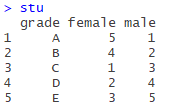is a data box that doesn’t mean anything, but what you would expect, is the distribution of scores by sex.
Variables of the female and male is said above is contained in the variable name variable, female and male should be “gender” the variable values of the variables, the number of the following variable names (or attribute name) should be the “number”, we need to keep the original grade a list below, get rid of the female and male two columns, increase sex and count two columns, values with the original table corresponding to the up respectively, using the gather function:

``gather(stu, gender, count,-grade)``

The first parameter is the original data STU, the second and third parameters are key-value pairs (gender, number of people), and the fourth parameter is subtracting (remove the grade column, only the remaining two columns are transposed).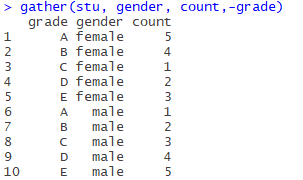If you look at the two columns in the original table, they correspond like this:
(female, 5), (female, 4), (female, 1), (female, 2), (female, 3)
(male, 1), (male, 2), (male, 3), (male, 4), (male, 5),
The original variable name (attribute name) is used as the key and the variable value as the value.
Now we can continue with the normal statistical analysis.
separate()
Separate the data in a variable that contains two variables. (The name of an attribute “Gather” is a variable.)
Separate (), for example
Construct a new data box Stu2:

``````stu2<-data.frame(grade=c("A","B","C","D","E"),
female_1=c(5, 4, 1, 2, 3), male_1=c(1, 2, 3, 4, 5),
female_2=c(4, 5, 1, 2, 3), male_2=c(0, 2, 3, 4, 6))``````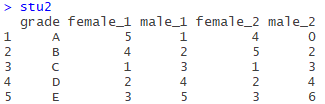is similar to stU above, with 1 and 2 after sex denoting classes
So let’s just use the Gather function and transpose:

``stu2_new<-gather(stu2,gender_class,count,-grade)``

No, just like above, the result is as follows: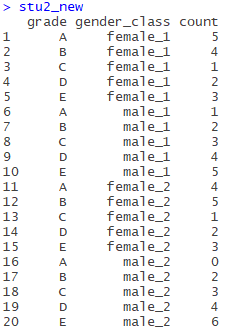While this table is still not a standard two-dimensional table, we have found a column (gender_class) with values containing multiple attributes (variables), separated by separate(), which is used as follows:
Separate (Data, Col, into, SEP (= regular expression), remove =TRUE,convert = FALSE, extra = “warn”, Fill = “warn”…)
The first parameter puts the data box to be separated;
The second argument puts the column to be separated;
The third parameter is the column (which must be multiple) of the split variable, represented by a vector;
The fourth argument is a delimiter, denoted by a regular expression, or a number, denoted by which digit it is separated from (in the documentation:
If character, is interpreted as a regular expression. The default value is a regular expression that matches any sequence of non-alphanumeric values.
If numeric, interpreted as positions to split at. Positive values start at 1 at the far-left of the string; Negative value start at-1 at the far right of the string. The length of sep should be one less than into.)
The following parameters are not clear, you can see the documentation
Now all we need to do is separate the gender_class column:

``separate(stu2_new,gender_class,c("gender","class"))``

Note that the third parameter is a vector, denoted by c(), and the fourth parameter should be “_”, omitted here (may be underlined is the default separator?).
The results are as follows: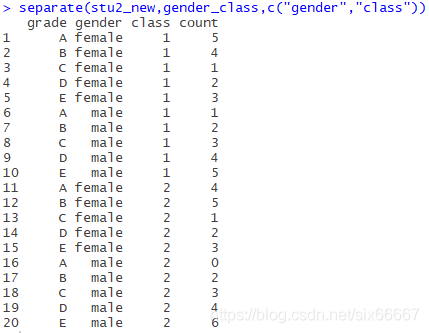spread()
Spread is used to extend the table to separate the values of a column (key-value pairs) into multiple columns.
spread(data, key, value, fill = NA, convert = FALSE, drop =TRUE, sep = NULL)
Key is the name of the original column (variable name), and value is what the value of those columns should be (which column of the original table should be filled).
So let’s go straight to the example
The spread (), for example
Construct data box Stu3:

``````name<-rep(c("Sally","Jeff","Roger","Karen","Brain"),c(2,2,2,2,2))
test<-rep(c("midterm","final"),5)
class1<-c("A","C",NA,NA,NA,NA,NA,NA,"B","B")
class2<-c(NA,NA,"D","E","C","A",NA,NA,NA,NA)
class3<-c("B","C",NA,NA,NA,NA,"C","C",NA,NA)
class4<-c(NA,NA,"A","C",NA,NA,"A","A",NA,NA)
class5<-c(NA,NA,NA,NA,"B","A",NA,NA,"A","C")
stu3<-data.frame(name,test,class1,class2,class3,class4,class5)``````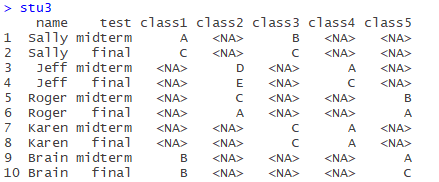There are 5 courses in total. Each student chooses 2 courses and lists the mid-term and final grades.
Obviously, the original table is dirty data, and the header contains the variable (class1-5), so use the Gather function first. Note that there are many missing values, so you can use the na.rm=TRUE parameter above to automatically remove records with missing values (a record is a row) :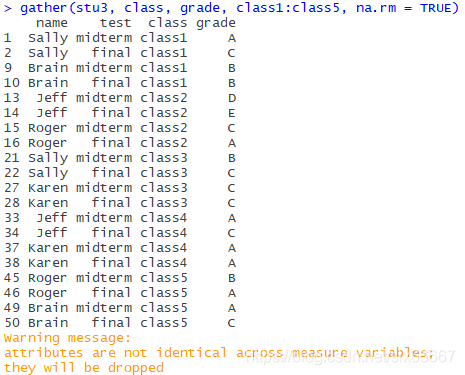If I didn’t write na.rm=TRUE, it would look like this: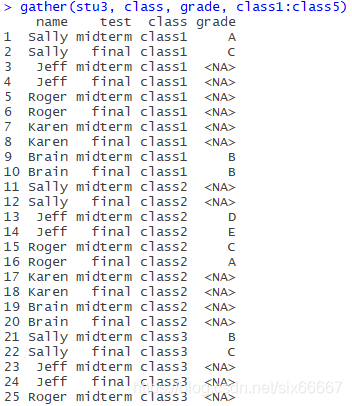(Incomplete)
It is meaningless to analyze the “NA” score of students who have not selected courses, so records with missing values should be discarded in this case.
Now this table looks very neat, but everyone has four records, in which the values of test and grade are different for each course, the names and courses are the same, and most of the time, we need to carry out statistical analysis on mid-term and final scores respectively, so this table is not conducive to classification statistics.
The test is midterm and final with the spread function, and the values of these two columns are the results of the two courses I chose.
Again, the second argument is the column name of the column to be split, and the third argument is the column name of which column the value of the expanded column should come from in the original table.

``````stu3_new<-gather(stu3, class, grade, class1:class5, na.rm = TRUE)
spread(stu3_new,test,grade)``````

The results are as follows: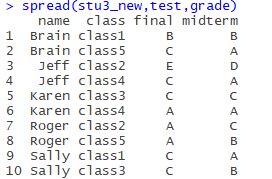Now that you have a very neat table with only 10 pieces of data, it’s much easier to process.
Finally, the class column is now a little redundant, and it’s a little more neat to just put Numbers in, use the parse_number() in the readr package to pull out the number(with the addition of dplyr’s mutate function), and let out the code:

``````install.packages("dplyr")
install.packages("readr")
library(readr)
library(dplyr)
mutate(spread(stu3_new,test,grade),class=parse_number(class))``````

Final results: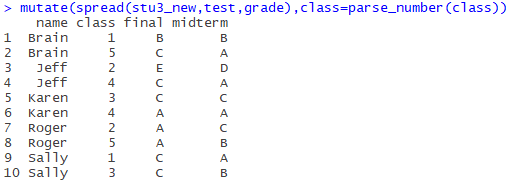Isn’t neat very good-looking!! (* ╹ del ╹ *)